Examples

Chapter 7 Class 11 Permutations and Combinations
Serial order wise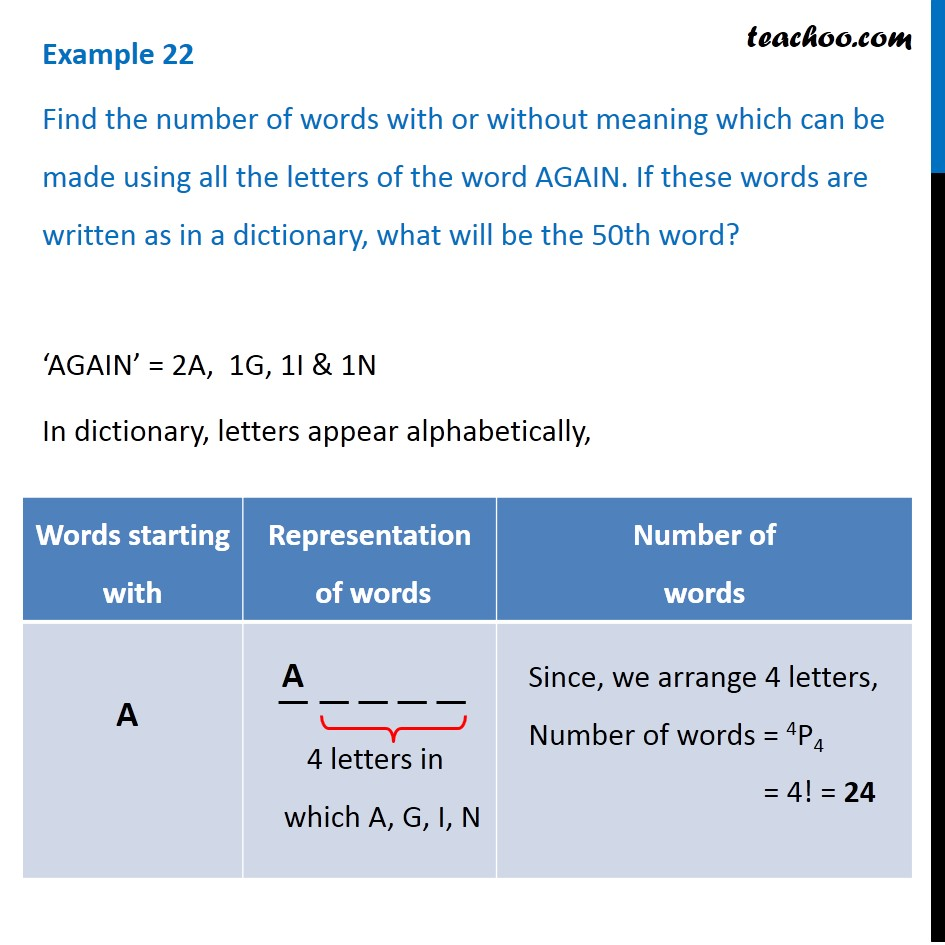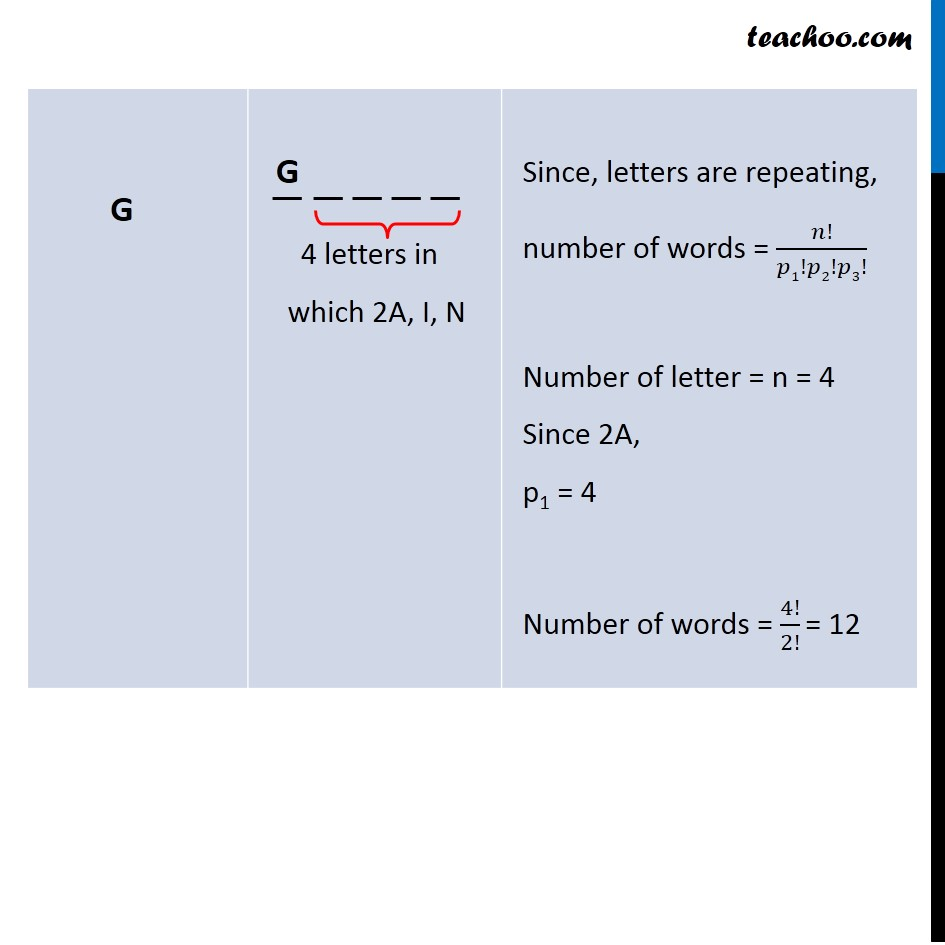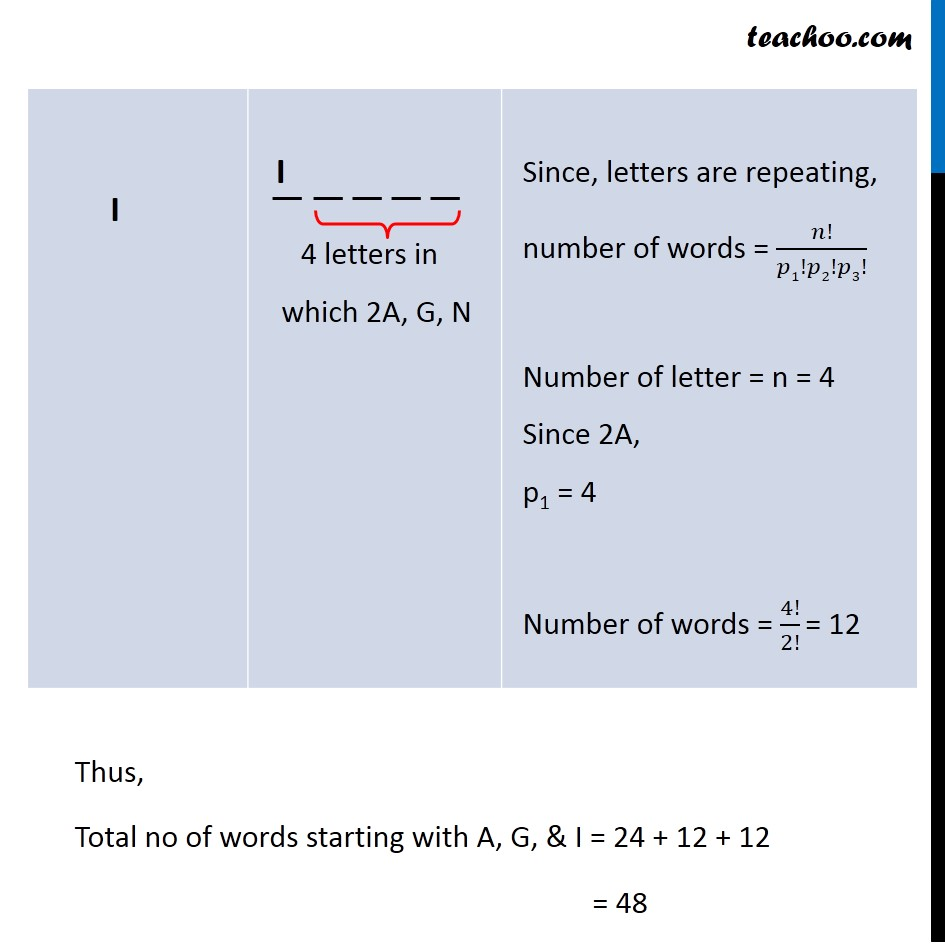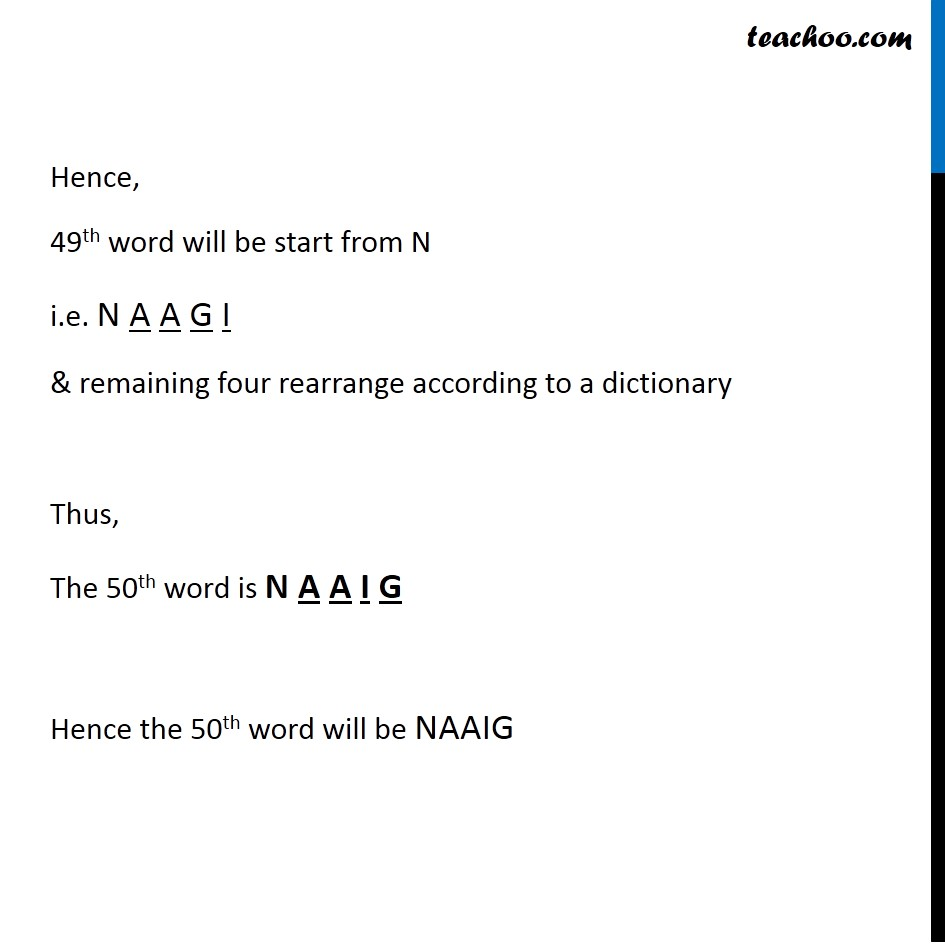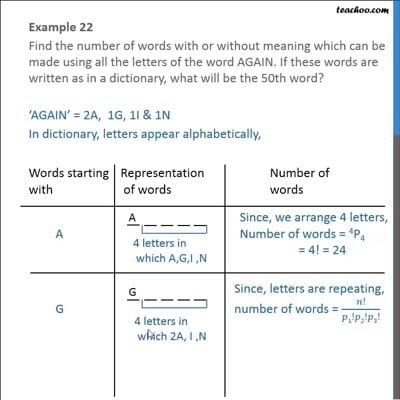This video is only available for Teachoo black users

Get live Maths 1-on-1 Classs - Class 6 to 12

### Transcript

Example 22 Find the number of words with or without meaning which can be made using all the letters of the word AGAIN. If these words are written as in a dictionary, what will be the 50th word? ‘AGAIN’ = 2A, 1G, 1I & 1N In dictionary, letters appear alphabetically, 4 letters in which A, G, I, N Since, we arrange 4 letters, Number of words = 4P4 = 4! = 24 4 letters in which 2A, I, N Since, letters are repeating, number of words = 𝑛!/𝑝1!𝑝2!𝑝3! Number of letter = n = 4 Since 2A, p1 = 4 Number of words = 4!/2! = 12 4 letters in which 2A, G, N Since, letters are repeating, number of words = 𝑛!/𝑝1!𝑝2!𝑝3! Number of letter = n = 4 Since 2A, p1 = 4 Number of words = 4!/2! = 12 Thus, Total no of words starting with A, G, & I = 24 + 12 + 12 = 48 Hence, 49th word will be start from N i.e. N A A G I & remaining four rearrange according to a dictionary Thus, The 50th word is N A A I G Hence the 50th word will be NAAIG